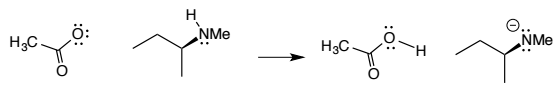Problem: For the transformation shown below, assuming it is a reversible transformation, which of the following would be correct for the equilibrium constant?a) K &gt; 1b) K &lt; 1c) K = 1d) K = 0

FREE Expert Solution
94% (228 ratings)
Problem Details

For the transformation shown below, assuming it is a reversible transformation, which of the following would be correct for the equilibrium constant?

a) K > 1

b) K < 1

c) K = 1

d) K = 0